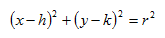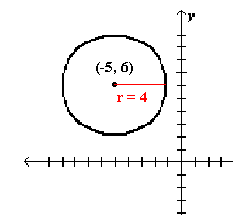Circles

A circle is the set of all points in a plane that are the same distance from a given point. The point is known as the center of the circle, and the distance is known as the radius. In order to graph a circle, the center and radius must be known. If the equation of the circle is given in standard form, the graph is fairly simple to create. The standard form of the equation of a circle is:(h, k) is the center of the circle

r is the length of the radius

Example Graph (x+5)2 + (y-6)2 = 16.

Step 1 . Identify the center and radius.

center (-5, 6) radius = 4

Step 2. Graph the circle.